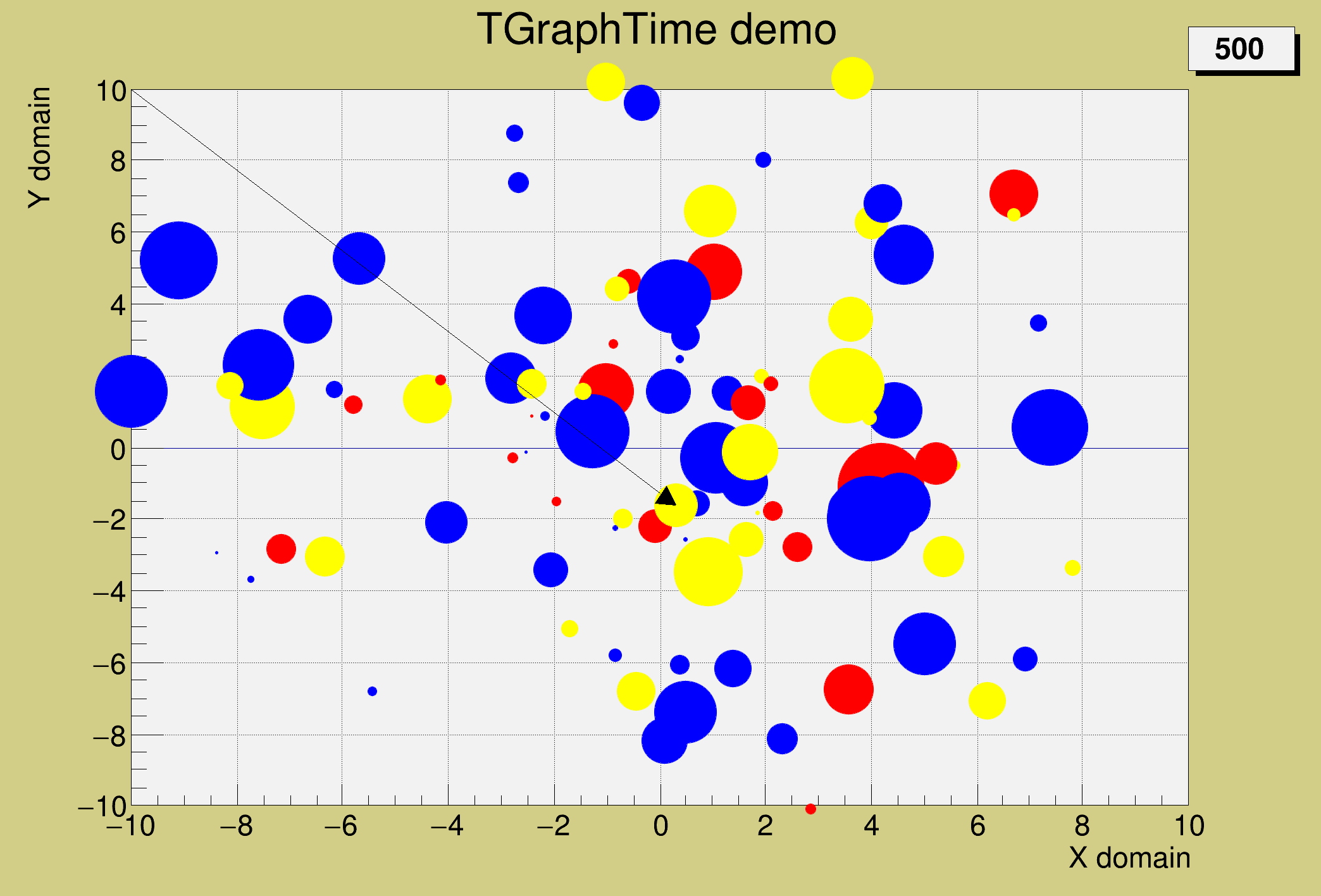ROOT   Reference Guidegtime.C File Reference

## Detailed Description#include "TCanvas.h"
#include "TRandom3.h"
#include "TMath.h"
#include "TText.h"
#include "TArrow.h"
#include "TGraphTime.h"
#include "TROOT.h"
void gtime(Int_t nsteps = 500, Int_t np=100) {
if (np > 1000) np = 1000;
Int_t color;
Double_t rr, phi, dr, size;
Double_t xmin = -10, xmax = 10, ymin = -10, ymax = 10;
g->SetTitle("TGraphTime demo;X domain;Y domain");
Int_t i,s;
for (i=0;i<np;i++) { //calculate some object parameters
rr[i] = r.Uniform(0.1*xmax,0.2*xmax);
phi[i] = r.Uniform(0,2*TMath::Pi());
dr[i] = r.Uniform(0,1)*0.9*xmax/Double_t(nsteps);
Double_t rc = r.Rndm();
color[i] = kRed;
if (rc > 0.3) color[i] = kBlue;
if (rc > 0.7) color[i] = kYellow;
size[i] = r.Uniform(0.5,6);
}
for (s=0;s<nsteps;s++) { //fill the TGraphTime step by step
for (i=0;i<np;i++) {
Double_t newr = rr[i]+dr[i]*s;
Double_t newsize = 0.2+size[i]*TMath::Abs(TMath::Sin(newr+10));
Double_t newphi = phi[i] + 0.01*s;
Double_t xx = newr*TMath::Cos(newphi);
Double_t yy = newr*TMath::Sin(newphi);
TMarker *m = new TMarker(xx,yy,20);
m->SetMarkerColor(color[i]);
m->SetMarkerSize(newsize);
}
}
g->Draw();
//save object to a file
TFile f("gtime.root","recreate");
g->Write("g");
//to view this object in another session do
// TFile f("gtime.root");
// g.Draw();
}
ROOT::R::TRInterface & r
Definition: Object.C:4
#define f(i)
Definition: RSha256.hxx:104
#define g(i)
Definition: RSha256.hxx:105
int Int_t
Definition: RtypesCore.h:45
double Double_t
Definition: RtypesCore.h:59
@ kRed
Definition: Rtypes.h:66
@ kBlue
Definition: Rtypes.h:66
@ kYellow
Definition: Rtypes.h:66
float xmin
Definition: THbookFile.cxx:95
float ymin
Definition: THbookFile.cxx:95
float xmax
Definition: THbookFile.cxx:95
float ymax
Definition: THbookFile.cxx:95
char * Form(const char *fmt,...)
Draw all kinds of Arrows.
Definition: TArrow.h:29
A ROOT file is a suite of consecutive data records (TKey instances) with a well defined format.
Definition: TFile.h:54
TGraphTime is used to draw a set of objects evolving with nsteps in time between tmin and tmax.
Definition: TGraphTime.h:29
Manages Markers.
Definition: TMarker.h:22
A Pave (see TPave) with a text centered in the Pave.
Definition: TPaveLabel.h:20
Random number generator class based on M.
Definition: TRandom3.h:27
static constexpr double s
Double_t Cos(Double_t)
Definition: TMath.h:643
constexpr Double_t Pi()
Definition: TMath.h:37
Double_t Sin(Double_t)
Definition: TMath.h:639
Short_t Abs(Short_t d)
Definition: TMathBase.h:120
auto * m
Definition: textangle.C:8

Definition in file gtime.C.